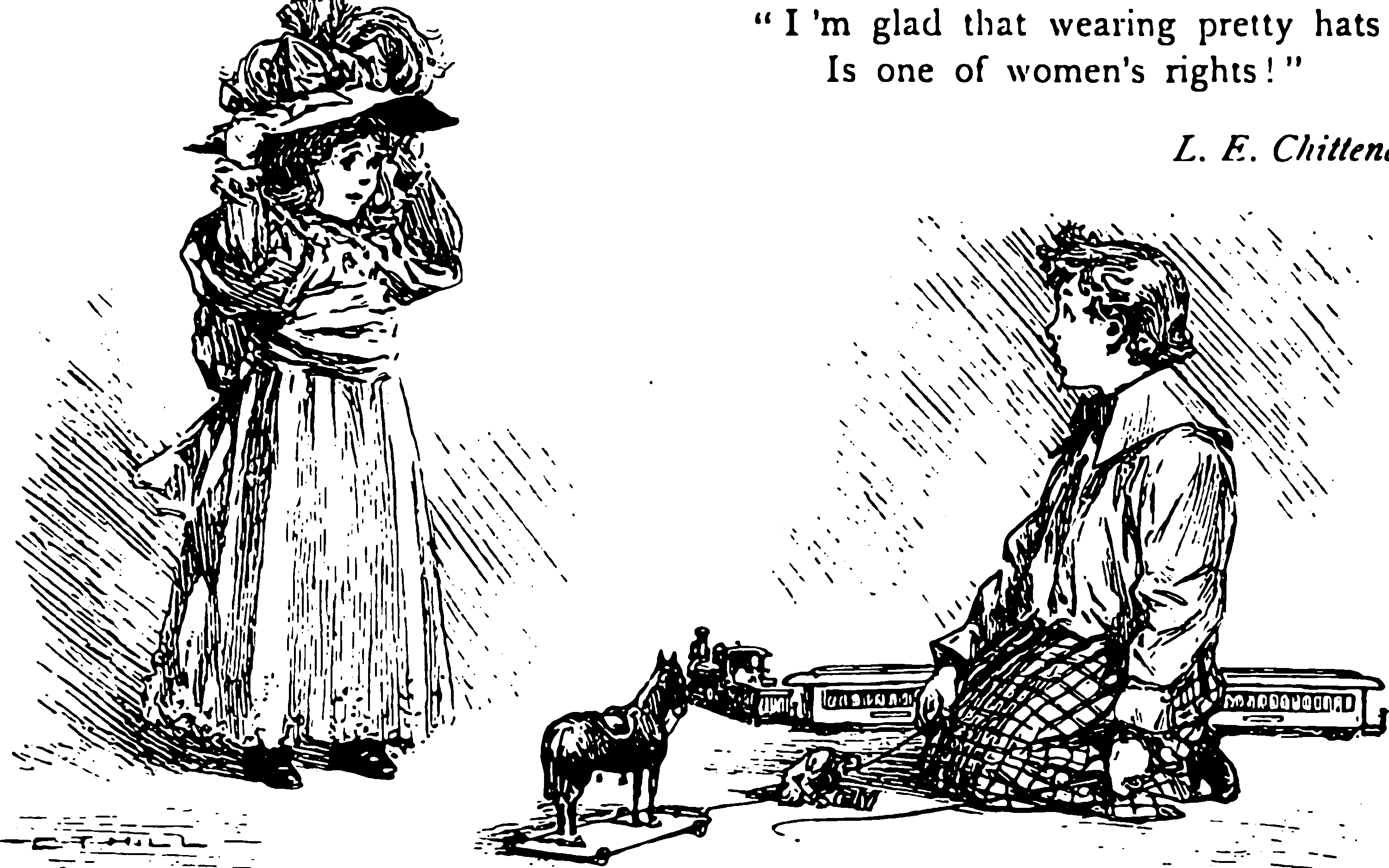# Probably Approximately CorrectA class of risk bounds, related in some way to concentration inequalities of statistical learning.

🏗 What is that way precisely?

Some historical notes: PAC learning was invented by Leslie Valiant in 1984, and it birthed a new subfield of computer science called computational learning theory and won Valiant some of computer science’s highest awards. Since then there have been numerous modifications of PAC learning, and also models that are entirely different from PAC learning. One other goal of learning theorists (as with computational complexity researchers) is to compare the power of different learning models.

## PAC-Bayes

Alquier (2021):

Aggregated predictors are obtained by making a set of basic predictors vote according to some weights, that is, to some probability distribution. Randomized predictors are obtained by sampling in a set of basic predictors, according to some prescribed probability distribution. Thus, aggregated and randomized predictors have in common that they are not defined by a minimization problem, but by a probability distribution on the set of predictors. In statistical learning theory, there is a set of tools designed to understand the generalization ability of such procedures: PAC-Bayesian or PAC-Bayes bounds. Since the original PAC-Bayes bounds of McAllester, these tools have been considerably improved in many directions (we will for example describe a simplified version of the localization technique of Catoni that was missed by the community, and later rediscovered as "mutual information bounds"). Very recently, PAC-Bayes bounds received a considerable attention: for example there was workshop on PAC-Bayes at NIPS 2017, "(Almost) 50 Shades of Bayesian Learning: PAC-Bayesian trends and insights", organized by B. Guedj, F. Bach and P. Germain. One of the reason of this recent success is the successful application of these bounds to neural networks by Dziugaite and Roy. An elementary introduction to PAC-Bayes theory is still missing. This is an attempt to provide such an introduction.I really struggled to find good illustrations for this.

### No comments yet. Why not leave one?

GitHub-flavored Markdown & a sane subset of HTML is supported.